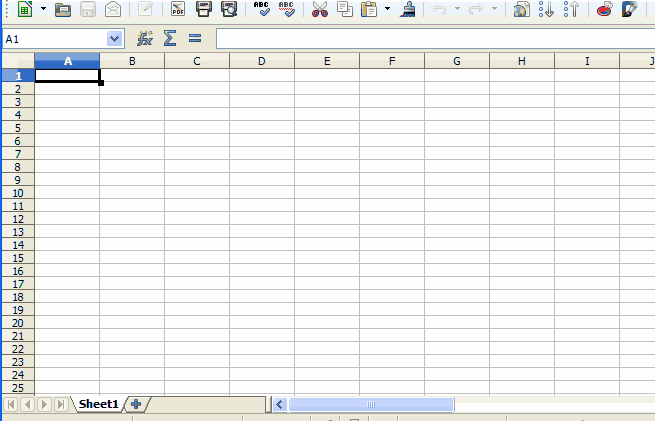# Calc, graph of y=2*x^2+5

Hello!
Please, can anyone help me to write the graph of the function y=2*x^2+5 in calc?
Do I need to make any table first, or is the formula enough?
How to get graph directly from the formula?

Many thanks!You will need to make a table first and then the graph as x-y-scatter.

Well, as the other answer demonstrates, you don’t need an xy-scatter plot/chart - a line chart works too.

Helloe, JohnSUN!
PLEASE, how did U get this animated?

Many thanks!!!

Oh, that’s easy enough. Fifty presses of PrintScreen button and this program

THANKS!!!

Hi JohnSUN, how did you make that trick? I meant Formula you put in \$B\$2 = 2X^2+5, the sheet turned back the value as if you type = 2[A2]^2 + 5.

@TingliChan Just open a spreadsheet document, choose Tools - Options - LibreOffice Calc - Calculate and check Automatically find column and row labels

Wow! Thank you.

I followed the steps in animation. Cells A2 and B2 being copied down A(n) went as expected, B(n) turned out constant which is 205. Why is that so?

The same behavior if you use the formula `=2*A2^2+5`, not `=2*X^2+5`?

Mm-hmm, =2*A2^2+5 was expected to be typed in B2 as a regular spreadsheet user. But I saw the illustration you gave out, it stunned me that a question came to my mind “Is there such a way to make things like that works out?” To me it seems like a new way to work things out. Very much thank you for your kind responses and patience to the newbie like I am.

It seems to me that yesterday, when I explained about “column labels”, everything worked correctly. Now I checked it, I see the same as you. If you save, close and open the document, the results are correct, but the Recalculate (F9) and Recalculate Hard (Ctrl+Shift+F9) have no effect. BTW, select (for example) B7 while it show 205 and press Ctrl+F2 (Function Wizard). Do you see? It show correct result 55. I think that it is bug of current version

@TingliChan I think I’ve found a workaround. Immediately after you stretch the formula down, copy the selected range Ctrl+C and immediately paste it on the same place Ctrl+V. This should work (but not the behavior we expect).

Save-Close-Reopen worked out as you explained. I tried save and File|Reload, this also did the trick too. But no luck with the Ctrl+C, Ctrl+V.

@JohnSUN Should we report the bug so the development team might have a look into the codes around this issue?

You can vote tdf#115654 (or tdf#127269?)